Figure 13.1 A plot with a linear gradient fill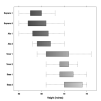R code
 Figure 13.2 A plot exported by gridSVG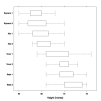R code
 Figure 13.3 A rectangle with a linear gradient fill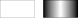R code
 Figure 13.4 Rectangles with radial gradient fills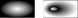R code
 Figure 13.5 Rectangles with pattern fills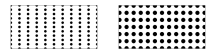R code
 Figure 13.6 A pattern fill based on several grobsR code
 Figure 13.7 Rectangles with filter effects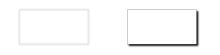R code
 Figure 13.8 Rectangles with a clipping path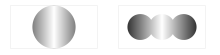R code
 Figure 13.9 Applying a black-and-white mask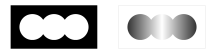R code
 Figure 13.10 Applying a grayscale mask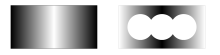R code
 Figure 13.11 A "bbox" gradient fill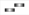R code
 Figure 13.12 A "coords" gradient fill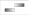R code
 Figure 13.13 A gradient fill defined within a viewport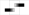R code
 Figure 13.14 An SVG file with a Google Font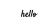R code